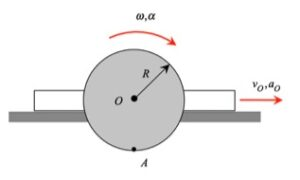# Homework H.2.BDiscussionThe velocity of A is always tangent to the path of A, and the acceleration of A always points inward on the path of A, as expected.

In solving the problem, write the velocity and acceleration of A referenced to the motion of the center of disk, O. Point O travels on a straight path, so its velocity and acceleration are always in the horizontal direction. Note that for a standard xy-coordinate system with x positive to the right and y positive upward, the angular velocity and angular acceleration of the disk are in the -z direction.